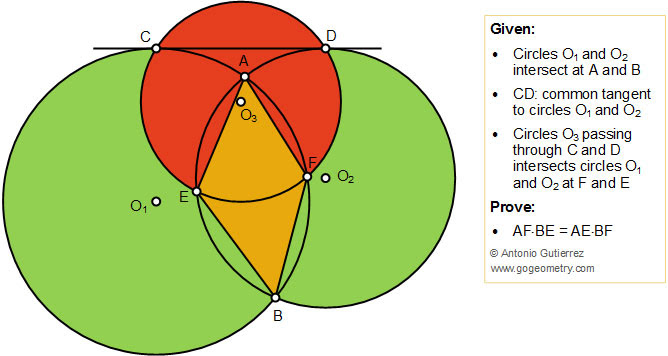## Friday, May 31, 2019

### Geometry Problem 1437: Intersecting Circles, Common Tangent, Equal Product

Geometry Problem. Post your solution in the comment box below.
Level: Mathematics Education, High School, Honors Geometry, College.

Details: Click on the figure below.#### 2 comments:

1.O1O2 ∩ CD=K
we 'll prove E,F,K in the same line (sorry for my english)
K ∈ Δ ,Δ ∩ (o2)=g,Δ ∩ (o1)=h
KO2/KO1=KD/KC(thales),KO2/KO1=r2/r1=KG/KH
so KD/KC=KG/KH and so DG // EH

Back to our problem we have that CDEF is Inscribed quadrilateral ,so DCF=DEF
EF∩(O1)=M , DCF=CMF so CMF=DEF so DE//CM
we can see with any Δ (K∈Δ) we'll have DG//CH so if DE//CM we'll have E and M ∈ Δ or
K,F,E,M ∈ Δ

KA ∩ (c2)=N,KB ∩ (c2)=P
we have AFMN is Inscribed quadrilateral so ΔKFA congruent with ΔKNM
so AF/MN=KA/KM(1)
just like that BF/MP=KB/KM(2)
(1):(2) so AF/BF=(KA/KB)*(MN/MP)(3)
and o2o1 perpendicular with AB ,K ∈ o2o1 so KA=KB (4)
from (3) and (4) we'll have AF/BF=MN/MP(a)

we can see that DA//CN,DE//CM so KA/KN=KE/KM or AE//MN (5)
just like that we also have BE//MP (6)
from (5) and (6) we'll have AE//BE=MN/MP(b)

And from (a) and (b) we'll have what we need

2.Bro,try to give a figure if the solution is very much constructive.